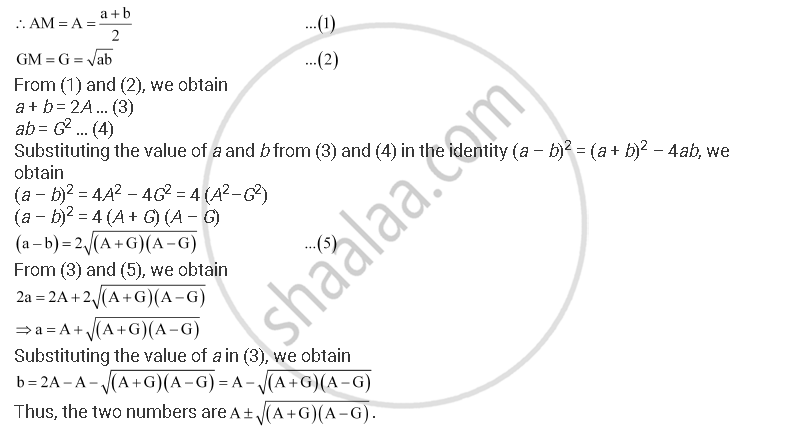CBSE (Arts) Class 11CBSE
Share

# If a and G Be A.M. and G.M., Respectively Between Two Positive Numbers, Prove that the Numbers Are A+- Sqrt((A+G)(A-g)) - CBSE (Arts) Class 11 - Mathematics

ConceptRelationship Between A.M. and G.M.

#### Question

If A and G be A.M. and G.M., respectively between two positive numbers, prove that the numbers are A+- sqrt((A+G)(A-G))

#### Solution

It is given that A and G are A.M. and G.M. between two positive numbers. Let these two positive numbers be a and b.Is there an error in this question or solution?

#### APPEARS IN

NCERT Solution for Mathematics Textbook for Class 11 (2018 to Current)
Chapter 9: Sequences and Series
Q: 29 | Page no. 193
Solution If a and G Be A.M. and G.M., Respectively Between Two Positive Numbers, Prove that the Numbers Are A+- Sqrt((A+G)(A-g)) Concept: Relationship Between A.M. and G.M..
S